# Complete Binary Tree

• Difficulty Level : Hard
• Last Updated : 24 Jun, 2022

We know a tree is a non-linear data structure. It has no limitation on the number of children. A binary tree has a limitation as any node of the tree has at most two children: a left and a right child.

## What is a Complete Binary Tree?

A complete binary tree is a special type of binary tree where all the levels of the tree are filled completely except the lowest level nodes which are filled from as left as possible.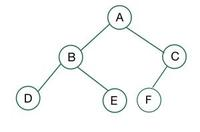Complete Binary Tree

## Some terminology of Complete Binary Tree:

• Root – Node in which no edge is coming from the parent. Example -node A
• Child – Node having some incoming edge is called child. Example – nodes B, H are the child of A and D respectively.
• Sibling – Nodes having the same parent are sibling. Example- J, K are siblings as they have the same parent E.
• Degree of a node – Number of children of a particular parent. Example- Degree of A is 2 and Degree of H is 1. Degree of L is 0.
• Internal/External nodes – Leaf nodes are external nodes and non leaf nodes are internal nodes.
• Level – Count nodes in a path to reach a destination node. Example- Level of node H is 3 as nodes A, D and H themselves form the path.
• Height – Number of edges to reach the destination node, Root is at height 0. Example – Height of node E is 2 as it has two edges from the root.

## Properties of Complete Binary Tree:

• A complete binary tree is said to be a proper binary tree where all leaves have the same depth.
• In a complete binary tree number of nodes at depth d is 2d
• In a  complete binary tree with n nodes height of the tree is log(n+1).
• All the levels except the last level are completely full.

## Perfect Binary Tree vs Complete Binary Tree:

A binary tree of height ‘h’ having the maximum number of nodes is a perfect binary tree.
For a given height h, the maximum number of nodes is 2h+1-1.

A complete binary tree of height h is a proper binary tree up to height h-1, and in the last level element are stored in left to right order.

Example 1: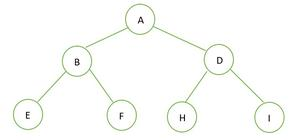A Binary Tree

The height of the given binary tree is 2 and the maximum number of nodes in that tree is n= 2h+1-1 =  22+1-1 =  23-1 = 7.
Hence we can conclude it is a perfect binary tree.
Now for a complete binary tree, It is full up to height h-1 i.e.; 1, and the last level elements are stored in left to right order. Hence it is a complete Binary tree also. Here is the representation of elements when stored in an array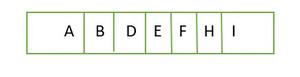Element stored in an array level by level

In the array, all the elements are stored continuously.

Example 2: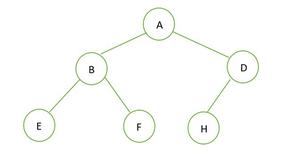A binary tree

Height of the given binary tree is 2 and the maximum number of nodes that should be there are 2h+1 – 1 = 22+1 – 1 = 23 – 1 = 7
But the number of nodes in the tree is 6. Hence it is not a perfect binary tree.
Now for a complete binary tree, It is full up to height h-1 i.e.; 1, and the last level element are stored in left to right order. Hence this is a complete binary tree. Store the element in an array and it will be like;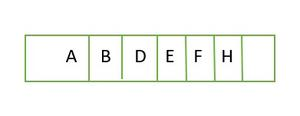Element stored in an array level by level

Example 3: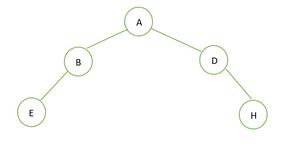A binary tree

The height of the binary tree is 2 and the maximum number of nodes that can be there is 7, but there are only 5 nodes hence it is not a perfect binary tree.
In case of a complete binary tree, we see that in the last level elements are not filled from left to right order. So it is not a complete binary tree.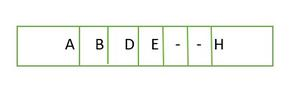Element stored in an array level by level

The elements in the array are not continuous.

## Full Binary Tree vs Complete Binary tree:

For a full binary tree, every node has either 2 children or 0 children.

Example 1: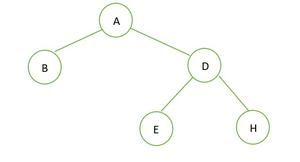A binary tree

In the given binary tree there is no node having degree 1, either 2 or 0 children for every node, hence it is a full binary tree.

For a complete binary tree, elements are stored in level by level and not from the leftmost side in the last level. Hence this is not a complete binary tree. The array representation is: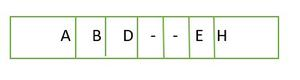Element stored in an array level by level

Example 2: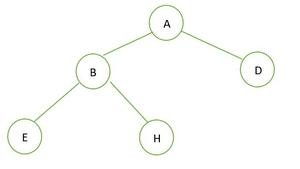A binary Tree

In the given binary tree there is no node having degree 1. Every node has a degree of either 2 or 0. Hence it is a full binary tree.

For a complete binary tree, elements are stored in a level by level manner and filled from the leftmost side of the last level. Hence this a complete binary tree. Below is the array representation of the tree: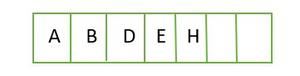Element stored in an array level by level

Example 3: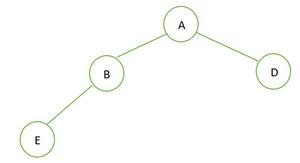A binary tree

In the given binary tree node B has degree 1 which violates the property of full binary tree hence it is not a full Binary tree

For a complete binary tree, elements are stored in level by level manner and filled from the leftmost side of the last level. Hence this is a complete binary tree. Array representation of the binary tree is: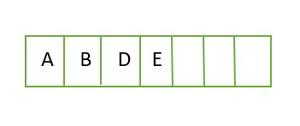Element stored in an array level by level

Example 4: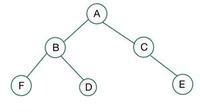a binary tree

In the given binary tree node C has degree 1 which violates the property of a full binary tree hence it is not a full Binary tree

For a complete binary tree, elements are stored in level by level manner and filled from the leftmost side of the last level. Here node E violates the condition. Hence this is not a complete binary tree

## Creation of Complete Binary Tree:

We know a complete binary tree is a tree in which except for the last level (say l)all the other level has (2l) nodes and the nodes are lined up from left to right side.
It can be represented using an array. If the parent is it index i so the left child is at 2i+1 and the right child is at 2i+2.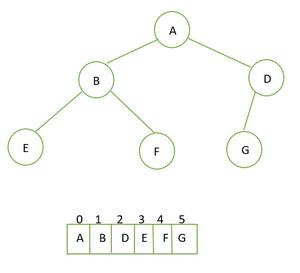Complete binary tree and its array representation

Algorithm:

For the creation of a Complete Binary Tree, we require a queue data structure to keep track of the inserted nodes.

Step 1: Initialize the root with a new node when the tree is empty.

Step 2: If the tree is not empty then get the front element

• If the front element does not have a left child then set the left child to a new node
• If the right child is not present set the right child as a new node

Step 3: If the node has both the children then pop it from the queue.

Step 4: Enqueue the new data.

Illustration:

Consider the below array:

1. The 1st element will the root (value at index = 0)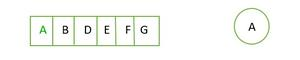A is taken as root

2. The next element (at index = 1) will be left and third element (index = 2) will be right child of root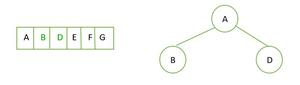B as left child and D as right child

3. fourth (index = 3) and fifth element (index = 4) will be the left and right child of B node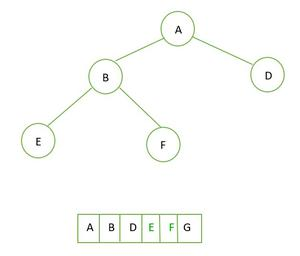E and F are left and right child of B

4. Next element (index = 5) will be left child of the node D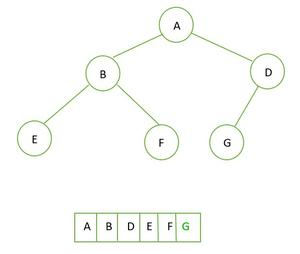G is made left child of D node

This is how complete binary tree is created.

Implementation: For the implementation of building a Complete Binary Tree from level order traversal is given in this post.

## Application of the Complete binary tree:

• Heap Sort
• Heap sort-based data structure

Check if a given binary tree is complete or not: Follow this post to check if the given binary tree is complete or not.

My Personal Notes arrow_drop_up
Recommended Articles
Page :